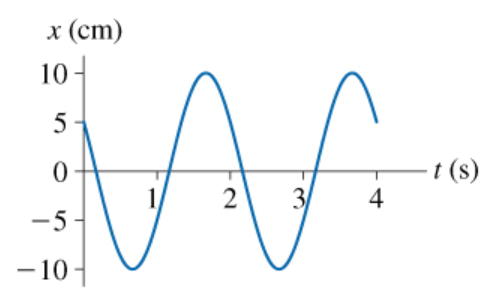# Problem: 1. What is the amplitude of the oscillation shown in (Figure 1) ?Express your answer using two significant figures2. What is the frequency of the oscillation shown in the figure?Express your answer using two significant figures.

###### FREE Expert Solution

1.

Amplitude is the maximum displacement from the equilibrium position.

93% (11 ratings)###### Problem Details1. What is the amplitude of the oscillation shown in (Figure 1) ?

2. What is the frequency of the oscillation shown in the figure?